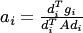Originally Conjugate Gradient method was developed for solving certain systems of linear equations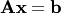where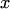is a known vector,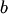is unknown and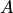is a square, symmetric, positive-definite matrix. Solving this equation is equivalent to finding minimum of a corresponding square function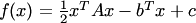This method is very similar to Gradient Descent in the sense that from the initial point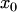we choose a vector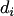which will take us to the next point, where the objective function will decrease. The above is repeated until we reach function minimum, i.e. gradient becomes zero.

Unlike Gradient Descent the direction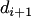is not set to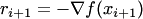, is chosen such that the next directionis conjugate towith respect to Hessian matrix.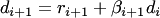A few approaches exist for calculating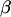. The most popular one are ones by Polak-Ribiere(1) and Fletcher-Reeves(2)

1.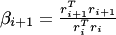2.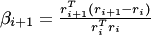So the algorithm looks like this:

1. Initializeto a random value
2. Initialize direction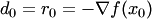3. Perform a line search: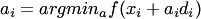4. Move to the next point: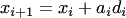5. Calculate gradient in next point:6. Calculate new direction:7. If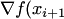is not small enough go to 3

Line search on step 3 can be replaced with an explicit solution, in cases where we can calculate Hessian matrixof our objective function. In this case we will get